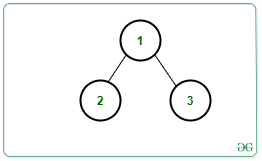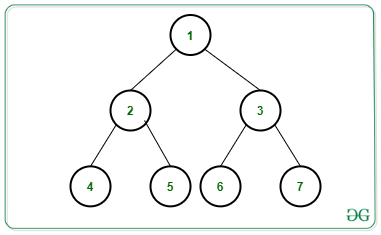GeeksforGeeks App
Open AppBrowser
Continue

# Count root to leaf paths having exactly K distinct nodes in a Binary Tree

Given a Binary Tree consisting of N nodes rooted at 1, an integer K and an array arr[] consisting of values assigned to each node, the task is to count the number of root to leaf paths having exactly K distinct nodes in the given Binary Tree.

Examples:

Input: N = 3, Edges[][] = {{1, 2}, {1, 3}}, arr[] = {3, 3, 2}, K = 2, Below is the given Tree:Output: 1
Explanation:
There exists only 1 distinct path i.e., Path 1 -> 3 contains 2 distinct nodes.

Input: N = 7, Edges[][] = {{1, 2}, {1, 3}, {2, 4}, {2, 5}, {3, 6}, {3, 7}}, arr[] = {2, 2, 2, 2, 3, 5, 2}, K = 1, Below is the given Tree:Output: 2
Explanation:
There exists only 2 distinct paths containing 1 distinct node:
1) Paths 1 -> 2 -> 4,
2) Paths 1 -> 3 -> 7

Naive Approach: The simplest approach is to generate all possible paths from the root to the leaf nodes and for each path, check if it contains K distinct nodes or not. Finally, print the count of such paths.

Time Complexity: O(N * H2), where H denotes the height of the tree.
Auxiliary Space: O(N);

Efficient Approach: The idea is to use Preorder Traversal and a Map to count the distinct node in the path from the root to the current node. Follow the below steps to solve the problem:

• Initialize a variable distinct_nodes as 0 to store the count of the distinct node from the root to the current node and ans as 0 to store the total distinct root to leaf paths having K distinct node.
• Perform Preorder Traversal in the given binary tree and store the count of the distinct node from root to the current node in the map M.
• Whenever a node occurs for the first time on a path, increase the count of distinct nodes by 1.
• If the count of distinct nodes on a path becomes greater than K return to the parent node of the current node.
• Otherwise, continue to visit the children of the current node incrementing the frequency of the current node value by 1.
• In the above step, increment ans by 1 if the count of distinct nodes on that root to leaf path is exactly equal to K.
• After the above steps print the value of ans as the resultant count.

Below is the implementation of the above approach:

## C++

 `// C++ program for the above approach``#include ``using` `namespace` `std;` `// Structure of a Tree Node``struct` `Node {``    ``int` `key;``    ``Node *left, *right;``};` `// Function to create new tree node``Node* newNode(``int` `key)``{``    ``Node* temp = ``new` `Node;``    ``temp->key = key;``    ``temp->left = temp->right = NULL;``    ``return` `temp;``}` `// Function to count all root to leaf``// paths having K distinct nodes``void` `findkDistinctNodePaths(``    ``Node* root, unordered_map<``int``, ``int``> freq,``    ``int` `distinct_nodes, ``int` `k, ``int``& ans)``{``    ``// If current node is null``    ``if` `(root == NULL)``        ``return``;` `    ``// Update count of distinct nodes``    ``if` `(freq[root->key] == 0)``        ``distinct_nodes++;` `    ``// If count > k then return to``    ``// the parent node``    ``if` `(distinct_nodes > k)``        ``return``;` `    ``// Update frequency of current node``    ``freq[root->key]++;` `    ``// Go to the left subtree``    ``findkDistinctNodePaths(root->left,``                           ``freq,``                           ``distinct_nodes,``                           ``k, ans);` `    ``// Go to the right subtree``    ``findkDistinctNodePaths(root->right,``                           ``freq,``                           ``distinct_nodes,``                           ``k, ans);` `    ``// If current node is leaf node``    ``if` `(root->left == NULL``        ``&& root->right == NULL) {` `        ``// If count of distinct node``        ``// is same as K, increment ans``        ``if` `(distinct_nodes == k)``            ``ans++;``    ``}``}` `// Function to find count of root to``// leaf paths having K distinct node``void` `printkDistinctNodePaths(Node* root,``                             ``int` `k)``{``    ``// Initialize unordered map``    ``unordered_map<``int``, ``int``> freq;` `    ``// Stores count of distinct node``    ``int` `distinct_nodes = 0;` `    ``// Stores total count of nodes``    ``int` `ans = 0;` `    ``// Perform Preorder Traversal``    ``findkDistinctNodePaths(root, freq,``                           ``distinct_nodes,``                           ``k, ans);` `    ``// Print the final count``    ``cout << ans;``}` `// Driver Code``int` `main()``{``    ``/*         2``             ``/   \``            ``/     \``           ``1       3``          ``/ \     /  \``         ``/   \   /    \``        ``4     2 -5     3``    ``*/` `    ``// Given Binary Tree``    ``Node* root = newNode(2);``    ``root->left = newNode(1);``    ``root->right = newNode(3);``    ``root->left->left = newNode(4);``    ``root->left->right = newNode(2);``    ``root->right->left = newNode(-5);``    ``root->right->right = newNode(3);` `    ``// Given K``    ``int` `K = 2;` `    ``// Function Call``    ``printkDistinctNodePaths(root, K);` `    ``return` `0;``}`

## Java

 `// Java program for the``// above approach``import` `java.util.*;``class` `GFG{` `// Structure of a``// Tree Node``static` `class` `Node``{``  ``int` `key;``  ``Node left, right;``};``  ` `static` `int` `ans;``  ` `// Function to create``// new tree node``static` `Node newNode(``int` `key)``{``  ``Node temp = ``new` `Node();``  ``temp.key = key;``  ``temp.left = temp.right = ``null``;``  ``return` `temp;``}` `// Function to count all root``// to leaf paths having K``// distinct nodes``static` `void` `findkDistinctNodePaths(Node root,``                                   ``HashMap freq,``                                   ``int` `distinct_nodes,``                                   ``int` `k)``{``  ``// If current node is null``  ``if` `(root == ``null``)``    ``return``;` `  ``// Update count of distinct nodes``  ``if` `(!freq.containsKey(root.key))``    ``distinct_nodes++;` `  ``// If count > k then return``  ``// to the parent node``  ``if` `(distinct_nodes > k)``    ``return``;` `  ``// Update frequency of``  ``// current node``  ``if``(freq.containsKey(root.key))``  ``{``    ``freq.put(root.key,``    ``freq.get(root.key) + ``1``);``  ``}``  ``else``  ``{``    ``freq.put(root.key, ``1``);``  ``}` `  ``// Go to the left subtree``  ``findkDistinctNodePaths(root.left, freq,``                         ``distinct_nodes, k);` `  ``// Go to the right subtree``  ``findkDistinctNodePaths(root.right, freq,``                         ``distinct_nodes, k);` `  ``// If current node is``  ``// leaf node``  ``if` `(root.left == ``null` `&&``      ``root.right == ``null``)``  ``{``    ``// If count of distinct node``    ``// is same as K, increment ans``    ``if` `(distinct_nodes == k)``      ``ans++;``  ``}``}` `// Function to find count of root to``// leaf paths having K distinct node``static` `void` `printkDistinctNodePaths(Node root,``                                    ``int` `k)``{``  ``// Initialize unordered map``  ``HashMap freq = ``new` `HashMap<>();` `  ``// Stores count of``  ``// distinct node``  ``int` `distinct_nodes = ``0``;` `  ``// Stores total``  ``// count of nodes``  ``ans = ``0``;` `  ``// Perform Preorder Traversal``  ``findkDistinctNodePaths(root, freq,``                         ``distinct_nodes, k);` `  ``// Print the final count``  ``System.out.print(ans);``}` `// Driver Code``public` `static` `void` `main(String[] args)``{``  ``/*           2``             ``/   \``            ``/     \``           ``1       3``          ``/ \     /  \``         ``/   \   /    \``        ``4     2 -5     3``    ``*/` `  ``// Given Binary Tree``  ``Node root = newNode(``2``);``  ``root.left = newNode(``1``);``  ``root.right = newNode(``3``);``  ``root.left.left = newNode(``4``);``  ``root.left.right = newNode(``2``);``  ``root.right.left = newNode(-``5``);``  ``root.right.right = newNode(``3``);` `  ``// Given K``  ``int` `K = ``2``;` `  ``// Function Call``  ``printkDistinctNodePaths(root, K);``}``}` `// This code is contributed by gauravrajput1`

## Python3

 `# Python3 program for the above approach` `# Structure of a Tree Node``class` `newNode:``    ` `    ``def` `__init__(``self``, key):``        ` `        ``self``.key ``=` `key``        ``self``.left ``=` `None``        ``self``.right ``=` `None` `ans ``=` `0` `# Function to count all root to leaf``# paths having K distinct nodes``def` `findkDistinctNodePaths(root, freq,``                           ``distinct_nodes, k):``                               ` `    ``global` `ans``    ` `    ``# If current node is None``    ``if` `(root ``=``=` `None``):``        ``return` `    ``# Update count of distinct nodes``    ``if` `(root.key ``not` `in` `freq):``        ``distinct_nodes ``+``=` `1` `    ``# If count > k then return to``    ``# the parent node``    ``if` `(distinct_nodes > k):``        ``return` `    ``# Update frequency of current node``    ``if` `(root.key ``in` `freq):``        ``freq[root.key] ``+``=` `1``    ``else``:``        ``freq[root.key] ``=` `freq.get(root.key, ``0``) ``+` `1` `    ``# Go to the left subtree``    ``findkDistinctNodePaths(root.left, freq,``                           ``distinct_nodes, k)` `    ``# Go to the right subtree``    ``findkDistinctNodePaths(root.right, freq,``                           ``distinct_nodes, k)` `    ``# If current node is leaf node``    ``if` `(root.left ``=``=` `None` `and``       ``root.right ``=``=` `None``):``        ` `        ``# If count of distinct node``        ``# is same as K, increment ans``        ``if` `(distinct_nodes ``=``=` `k):``            ``ans ``+``=` `1` `# Function to find count of root to``# leaf paths having K distinct node``def` `printkDistinctNodePaths(root, k):``    ` `    ``global` `ans``    ` `    ``# Initialize unordered map``    ``freq ``=` `{}` `    ``# Stores count of distinct node``    ``distinct_nodes ``=` `0` `    ``# Perform Preorder Traversal``    ``findkDistinctNodePaths(root, freq,``                           ``distinct_nodes, k)` `    ``# Print the final count``    ``print``(ans)` `# Driver Code``if` `__name__ ``=``=` `'__main__'``:``    ` `    ``'''        2``             ``/   \``            ``/     \``           ``1       3``          ``/ \     /  \``         ``/   \   /    \``        ``4     2 -5     3``    ``'''` `    ``# Given Binary Tree``    ``root ``=` `newNode(``2``)``    ``root.left ``=` `newNode(``1``)``    ``root.right ``=` `newNode(``3``)``    ``root.left.left ``=` `newNode(``4``)``    ``root.left.right ``=` `newNode(``2``)``    ``root.right.left ``=` `newNode(``-``5``)``    ``root.right.right ``=` `newNode(``3``)` `    ``# Given K``    ``K ``=` `2` `    ``# Function Call``    ``printkDistinctNodePaths(root, K)``    ` `# This code is contributed by SURENDRA_GANGWAR`

## C#

 `// C# program for the``// above approach``using` `System;``using` `System.Collections.Generic;` `class` `GFG{` `// Structure of a``// Tree Node``public` `class` `Node``{``  ``public` `int` `key;``  ``public` `Node left, right;``};``  ` `static` `int` `ans;``  ` `// Function to create``// new tree node``static` `Node newNode(``int` `key)``{``  ``Node temp = ``new` `Node();``  ``temp.key = key;``  ``temp.left = temp.right = ``null``;``  ``return` `temp;``}``  ` `// Function to count all root``// to leaf paths having K``// distinct nodes``static` `void` `findkDistinctNodePaths(Node root,``                                   ``Dictionary<``int``, ``int``> freq,``                                   ``int` `distinct_nodes,``                                   ``int` `k)``{``  ` `  ``// If current node is null``  ``if` `(root == ``null``)``    ``return``;` `  ``// Update count of distinct nodes``  ``if` `(!freq.ContainsKey(root.key))``    ``distinct_nodes++;` `  ``// If count > k then return``  ``// to the parent node``  ``if` `(distinct_nodes > k)``    ``return``;` `  ``// Update frequency of``  ``// current node``  ``if` `(freq.ContainsKey(root.key))``  ``{``    ``freq[root.key] = freq[root.key] + 1;``  ``}``  ``else``  ``{``    ``freq.Add(root.key, 1);``  ``}` `  ``// Go to the left subtree``  ``findkDistinctNodePaths(root.left, freq,``                         ``distinct_nodes, k);` `  ``// Go to the right subtree``  ``findkDistinctNodePaths(root.right, freq,``                         ``distinct_nodes, k);` `  ``// If current node is``  ``// leaf node``  ``if` `(root.left == ``null` `&&``      ``root.right == ``null``)``  ``{``    ` `    ``// If count of distinct node``    ``// is same as K, increment ans``    ``if` `(distinct_nodes == k)``      ``ans++;``  ``}``}` `// Function to find count of root to``// leaf paths having K distinct node``static` `void` `printkDistinctNodePaths(Node root,``                                    ``int` `k)``{``  ` `  ``// Initialize unordered map``  ``Dictionary<``int``,``             ``int``> freq = ``new` `Dictionary<``int``,``                                        ``int``>();``  ` `  ``// Stores count of``  ``// distinct node``  ``int` `distinct_nodes = 0;` `  ``// Stores total``  ``// count of nodes``  ``ans = 0;` `  ``// Perform Preorder Traversal``  ``findkDistinctNodePaths(root, freq,``                         ``distinct_nodes, k);` `  ``// Print the readonly count``  ``Console.Write(ans);``}` `// Driver Code``public` `static` `void` `Main(String[] args)``{``  ``/*           2``             ``/   \``            ``/     \``           ``1       3``          ``/ \     /  \``         ``/   \   /    \``        ``4     2 -5     3``    ``*/` `  ``// Given Binary Tree``  ``Node root = newNode(2);``  ``root.left = newNode(1);``  ``root.right = newNode(3);``  ``root.left.left = newNode(4);``  ``root.left.right = newNode(2);``  ``root.right.left = newNode(-5);``  ``root.right.right = newNode(3);` `  ``// Given K``  ``int` `K = 2;` `  ``// Function Call``  ``printkDistinctNodePaths(root, K);``}``}` `// This code is contributed by Princi Singh`

## Javascript

 ``

Output:

`2`

Time Complexity: O(N)
Auxiliary Space: O(N)

My Personal Notes arrow_drop_up